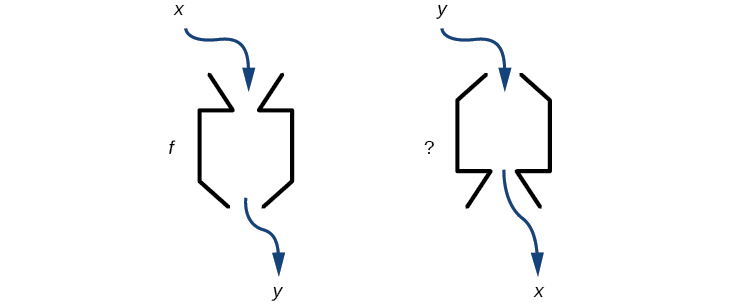3.7 Inverse functions

 Page 1 / 9
In this section, you will:
• Verify inverse functions.
• Determine the domain and range of an inverse function, and restrict the domain of a function to make it one-to-one.
• Find or evaluate the inverse of a function.
• Use the graph of a one-to-one function to graph its inverse function on the same axes.

A reversible heat pump is a climate-control system that is an air conditioner and a heater in a single device. Operated in one direction, it pumps heat out of a house to provide cooling. Operating in reverse, it pumps heat into the building from the outside, even in cool weather, to provide heating. As a heater, a heat pump is several times more efficient than conventional electrical resistance heating.

If some physical machines can run in two directions, we might ask whether some of the function “machines” we have been studying can also run backwards. [link] provides a visual representation of this question. In this section, we will consider the reverse nature of functions.Can a function “machine” operate in reverse?

Verifying that two functions are inverse functions

Suppose a fashion designer traveling to Milan for a fashion show wants to know what the temperature will be. He is not familiar with the Celsius scale. To get an idea of how temperature measurements are related, he asks his assistant, Betty, to convert 75 degrees Fahrenheit to degrees Celsius. She finds the formula

$C=\frac{5}{9}\left(F-32\right)$

and substitutes 75 for $\text{\hspace{0.17em}}F\text{\hspace{0.17em}}$ to calculate

$\frac{5}{9}\left(75-32\right)\approx 24\text{°C}$

Knowing that a comfortable 75 degrees Fahrenheit is about 24 degrees Celsius, he sends his assistant the week’s weather forecast from [link] for Milan, and asks her to convert all of the temperatures to degrees Fahrenheit.

At first, Betty considers using the formula she has already found to complete the conversions. After all, she knows her algebra, and can easily solve the equation for $\text{\hspace{0.17em}}F\text{\hspace{0.17em}}$ after substituting a value for $\text{\hspace{0.17em}}C.\text{\hspace{0.17em}}$ For example, to convert 26 degrees Celsius, she could write

$\begin{array}{ccc}\hfill 26& =& \frac{5}{9}\left(F-32\right)\hfill \\ \hfill 26\cdot \frac{9}{5}& =& F-32\hfill \\ \hfill F& =& 26\cdot \frac{9}{5}+32\approx 79\hfill \end{array}$

After considering this option for a moment, however, she realizes that solving the equation for each of the temperatures will be awfully tedious. She realizes that since evaluation is easier than solving, it would be much more convenient to have a different formula, one that takes the Celsius temperature and outputs the Fahrenheit temperature.

The formula for which Betty is searching corresponds to the idea of an inverse function , which is a function for which the input of the original function becomes the output of the inverse function and the output of the original function becomes the input of the inverse function.

Given a function $\text{\hspace{0.17em}}f\left(x\right),\text{\hspace{0.17em}}$ we represent its inverse as $\text{\hspace{0.17em}}{f}^{-1}\left(x\right),\text{\hspace{0.17em}}$ read as $\text{\hspace{0.17em}}“f\text{\hspace{0.17em}}$ inverse of $\text{\hspace{0.17em}}x.\text{”}\text{\hspace{0.17em}}$ The raised $\text{\hspace{0.17em}}-1\text{\hspace{0.17em}}$ is part of the notation. It is not an exponent; it does not imply a power of $\text{\hspace{0.17em}}-1\text{\hspace{0.17em}}$ . In other words, $\text{\hspace{0.17em}}{f}^{-1}\left(x\right)\text{\hspace{0.17em}}$ does not mean $\text{\hspace{0.17em}}\frac{1}{f\left(x\right)}\text{\hspace{0.17em}}$ because $\text{\hspace{0.17em}}\frac{1}{f\left(x\right)}\text{\hspace{0.17em}}$ is the reciprocal of $\text{\hspace{0.17em}}f\text{\hspace{0.17em}}$ and not the inverse.

The “exponent-like” notation comes from an analogy between function composition and multiplication: just as $\text{\hspace{0.17em}}{a}^{-1}a=1\text{\hspace{0.17em}}$ (1 is the identity element for multiplication) for any nonzero number $\text{\hspace{0.17em}}a,\text{\hspace{0.17em}}$ so $\text{\hspace{0.17em}}{f}^{-1}\circ f\text{\hspace{0.17em}}$ equals the identity function, that is,

sin^4+sin^2=1, prove that tan^2-tan^4+1=0
what is the formula used for this question? "Jamal wants to save \$54,000 for a down payment on a home. How much will he need to invest in an account with 8.2% APR, compounding daily, in order to reach his goal in 5 years?"
i don't need help solving it I just need a memory jogger please.
Kuz
A = P(1 + r/n) ^rt
Dale
how to solve an expression when equal to zero
its a very simple
Kavita
gave your expression then i solve
Kavita
Hy guys, I have a problem when it comes on solving equations and expressions, can you help me 😭😭
Thuli
Tomorrow its an revision on factorising and Simplifying...
Thuli
ok sent the quiz
kurash
send
Kavita
Hi
Masum
What is the value of log-1
Masum
the value of log1=0
Kavita
Log(-1)
Masum
What is the value of i^i
Masum
log -1 is 1.36
kurash
No
Masum
no I m right
Kavita
No sister.
Masum
no I m right
Kavita
tan20°×tan30°×tan45°×tan50°×tan60°×tan70°
jaldi batao
Joju
Find the value of x between 0degree and 360 degree which satisfy the equation 3sinx =tanx
what is sine?
what is the standard form of 1
1×10^0
Akugry
Evalute exponential functions
30
Shani
The sides of a triangle are three consecutive natural number numbers and it's largest angle is twice the smallest one. determine the sides of a triangle
Will be with you shortly
Inkoom
3, 4, 5 principle from geo? sounds like a 90 and 2 45's to me that my answer
Neese
answer is 2, 3, 4
Gaurav
prove that [a+b, b+c, c+a]= 2[a b c]
can't prove
Akugry
i can prove [a+b+b+c+c+a]=2[a+b+c]
this is simple
Akugry
hi
Stormzy
x exposant 4 + 4 x exposant 3 + 8 exposant 2 + 4 x + 1 = 0
x exposent4+4x exposent3+8x exposent2+4x+1=0
HERVE
How can I solve for a domain and a codomains in a given function?
ranges
EDWIN
Thank you I mean range sir.
Oliver
proof for set theory
don't you know?
Inkoom
find to nearest one decimal place of centimeter the length of an arc of circle of radius length 12.5cm and subtending of centeral angle 1.6rad
factoring polynomialByBy